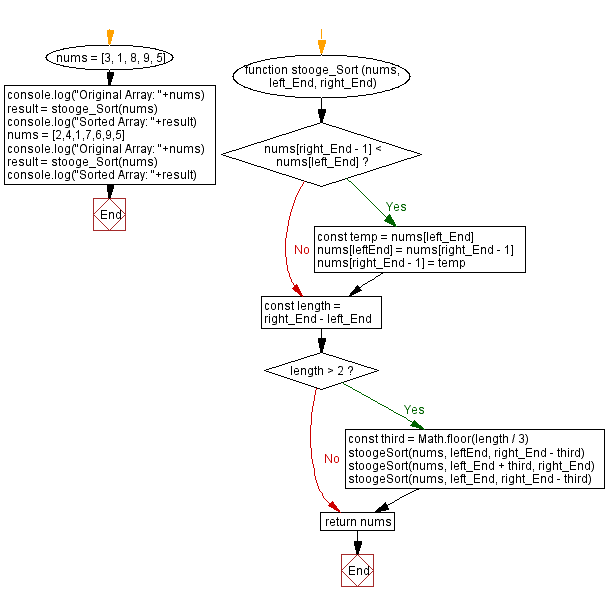# JavaScript Sorting Algorithm: Stooge sort

## JavaScript Sorting Algorithm: Exercise-31 with Solution

From Wikipedia –
Stooge sort is a recursive sorting algorithm. It is notable for its exceptionally bad time complexity of O(nlog 3 / log 1.5 ) = O(n2.7095...). The running time of the algorithm is thus slower compared to reasonable sorting algorithms, and is slower than bubble sort, a canonical example of a fairly inefficient sort. It is however more efficient than Slowsort. The name comes from The Three Stooges.

The algorithm is defined as follows:

• If the value at the start is larger than the value at the end, swap them.
• If there are 3 or more elements in the list, then:
Stooge sort the initial 2/3 of the list
Stooge sort the final 2/3 of the list
Stooge sort the initial 2/3 of the list again

Write a JavaScript program to sort a list of elements using the Stooge sort algorithm.

Sample Data: Original Array: [3, 1, 8, 9, 5]
Sorted Array: [1, 3, 5, 8, 9]
Original Array: [2, 4, 1, 7, 6, 9, 5]
Sorted Array: [1, 2, 4, 5, 6, 7, 9]

Sample Solution:

JavaScript Code:

``````function stooge_Sort (nums, left_End, right_End)
{
if (nums[right_End - 1] < nums[left_End])
{
const temp = nums[left_End]
nums[leftEnd] = nums[right_End - 1]
nums[right_End - 1] = temp
}
const length = right_End - left_End
if (length > 2)
{
const third = Math.floor(length / 3)
stoogeSort(nums, leftEnd, right_End - third)
stoogeSort(nums, left_End + third, right_End)
stoogeSort(nums, left_End, right_End - third)
}
return nums
}
nums = [3, 1, 8, 9, 5]
console.log("Original Array: "+nums)
result = stooge_Sort(nums)
console.log("Sorted Array: "+result)
nums = [2,4,1,7,6,9,5]
console.log("Original Array: "+nums)
result = stooge_Sort(nums)
console.log("Sorted Array: "+result)
```
```

Sample Output:

```"Original Array: 3,1,8,9,5"
"Sorted Array: 3,1,8,9,5"
"Original Array: 2,4,1,7,6,9,5"
"Sorted Array: 2,4,1,7,6,9,5"
```

Flowchart:Live Demo:

See the Pen searching-and-sorting-algorithm-exercise-31 by w3resource (@w3resource) on CodePen.

Improve this sample solution and post your code through Disqus

What is the difficulty level of this exercise?

Test your Programming skills with w3resource's quiz.

﻿